# Part 2: Scan Conversion on Line Generation Algorithm.

## Scan Conversion on Line Generation Algorithm

Scan Conversion defer  is a process of representing graphics objects a collection of pixels that is 1(on set) or 0 (offset). In general, a line consist of connecting two points. It is a basic  in computer graphics. In order to draw a line, you need two points between which you can draw a line.

### Scan Converting a Straight Line

A straight line may be defined by two endpoints & an equation. In fig the two endpoints are described by (x1,y1) and (x2,y2). The equation of the line is used to determine the x, y coordinates of all the points that lie between these two endpoints.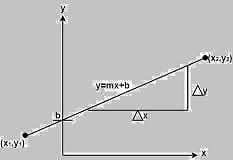Using the equation of a straight line, y = mx + b where m =& b = the y interrupt, we can find values of y by incrementing x from x =x1, to x = x2. By scan-converting these calculated x, y values, we represent the line as a sequence of pixels.

### Properties of Good Line Drawing Algorithm:

1. Line should appear Straight: We must appropriate the line by choosing addressable points close to it. If we choose well, the line will appear straight, if not, we shall produce crossed lines.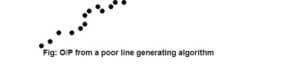The lines must be generated parallel or at 45° to the x and y-axes. Other lines cause a problem: a line segment through it starts and finishes at addressable points, may happen to pass through no another addressable points in between.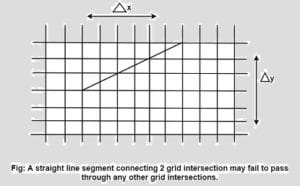2. Lines should terminate accurately: Unless lines are plotted accurately, they may terminate at the wrong place.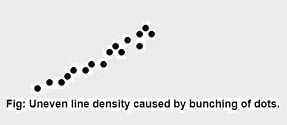3. Lines should have constant density: Line density is proportional to the no. of dots displayed divided by the length of the line. In order to maintain constant density, dots should be equally spaced.

4. Line density should be independent of line length and angle: This can be done by computing an approximating line-length estimate and to use a line-generation algorithm that keeps line density constant to within the accuracy of this estimate.

5. Line should be drawn rapidly: This computation should be performed by special-purpose hardware.

### Algorithm for line Drawing:

1. Direct use of line equation
2. DDA (Digital Differential Analyzer)
3. Bresenham’s Algorithm

### Direct use of line equation:

It is the simplest form of conversion. First of all scan P1 and P2 points. P1 has co-ordinates (x1‘,y1‘) and (x2‘ y2‘ ).

Then       m = (y2‘,y1‘)/( x2‘,x1‘) and b =If value of |m|≤1 for each integer value of x. But do not considerIf value of |m|>1 for each integer value of y. But do not considerExample: A line with starting point as (0, 0) and ending point (6, 18) is given. Calculate value of intermediate points and slope of line.

Solution: P1 (0,0) P7 (6,18)

x1=0
y1=0
x2=6
y2=18We know equation of line is
y =m x + b
y = 3x + b…………..equation (1)

put value of x from initial point in equation (1), i.e., (0, 0) x =0, y=0
0 = 3 x 0 + b
0 = b ⟹ b=0

put b = 0 in equation (1)
y = 3x + 0
y = 3x

Now calculate intermediate points

Let x = 1 ⟹ y = 3 x 1 ⟹ y = 3
Let x = 2 ⟹ y = 3 x 2 ⟹ y = 6
Let x = 3 ⟹ y = 3 x 3 ⟹ y = 9
Let x = 4 ⟹ y = 3 x 4 ⟹ y = 12
Let x = 5 ⟹ y = 3 x 5 ⟹ y = 15
Let x = 6 ⟹ y = 3 x 6 ⟹ y = 18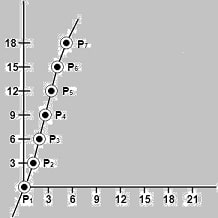So points are

P1 (0,0);  P2 (1,3) ; P3 (2,6); P4 (3,9); P5 (4,12); P6 (5,15); P7 (6,18)

### Algorithm for drawing line using equation:

Step1: Start Algorithm

Step2: Declare variables x1,x2,y1,y2,dx,dy,m,b,

Step3: Enter values of x1,x2,y1,y2.
The (x1,y1) are co-ordinates of a starting point of the line.
The (x2,y2) are co-ordinates of a ending point of the line.

Step4: Calculate dx = x2– x1

Step5: Calculate dy = y2-y1

Step6: Calculate m =Step7: Calculate b = y1-m* x1

Step8: Set (x, y) equal to starting point, i.e., lowest point and xendequal to largest value of x.

If dx < 0
then x = x2
y = y2
xend= x1
If dx > 0
then x = x1
y = y1
xend= x2

Step9: Check whether the complete line has been drawn if x=xend, stop

Step10: Plot a point at current (x, y) coordinates

Step11: Increment value of x, i.e., x = x+1

Step12: Compute next value of y from equation y = mx + b

Step13: Go to Step9.

### Program to draw a line using Line Slope Method

#include <graphics.h>
#include <stdlib.h>
#include <math.h>
#include <stdio.h>
#include <conio.h>
#include <iostream>

using namespace std;

class bresen
{
float x, y, x1, y1, x2, y2, dx, dy, m, c, xend;
public:
void get ();
void cal ();
};
int main ()
{
bresen b;
b.get ();
b.cal ();
getch ();
}
void bresen :: get ()
{
printf (“Enter start & end points”);
printf (“enter x1, y1, x2, y2\n”);
scanf (“%f%f%f%f”,&x1, &y1, &x2, &y2);
}
void bresen ::cal ()
{
/* request auto detection */
int gdriver = DETECT,gmode, errorcode;
/* initialize graphics and local variables */
initgraph (&gdriver, &gmode, ” “);
/* read result of initialization */
errorcode = graphresult();
if (errorcode != grOk) /* an error occurred */ /*an error occurred */
{
printf(“Graphics error: %s\n”, grapherrormsg(errorcode));
printf(“Press any key to halt:”);
getch ();
exit (1); /* terminate with an error code */
}
dx = x2-x1;
dy=y2-2*y1;
m = dy/dx;
c = y1 – (m * x1);
if (dx<0)
{
x=x2;
y=y2;
xend=x1;
}
else
{
x=x1;
y=y1;
xend=x2;
}
while (x<=xend)
{
putpixel (x, y, RED);
y++;
y=(x*x) +c;
}
}

OUTPUT:

Enter Starting and End Points
Enter (X1, Y1, X2, Y2)
200 100 300 200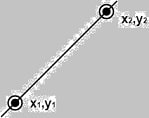In general, a line consist of connecting two points. It is a basic  in computer graphics. In order to draw a line, you need two points between which you can draw a line.

In the following three algorithms, we refer the one point of line as X0,Y0X0,Y0 and the second point of line as X1,Y1X1,Y1.

### DDA Algorithm(Digital Differential Analyzer )

DDA algorithm is the simple line generation algorithm which is detailed step by step here.

Step 1 − Get the input of two end points (X0,Y0)(X0,Y0) and (X1,Y1)(X1,Y1).

Step 2 − Calculate the difference between two end points.

dx = X1 - X0
dy = Y1 - Y0

Step 3 − Based on the calculated difference in step-2, you need to identify the number of steps to put pixel. If dx > dy, then you need more steps in x coordinate; otherwise in y coordinate.

if (absolute(dx) > absolute(dy))
Steps = absolute(dx);
else
Steps = absolute(dy);

Step 4 − Calculate the increment in x coordinate and y coordinate.

Xincrement = dx / (float) steps;
Yincrement = dy / (float) steps;


Step 5 − Put the pixel by successfully incrementing x and y coordinates accordingly and complete the drawing of the line.

for(int v=0; v < Steps; v++)
{
x = x + Xincrement;
y = y + Yincrement;
putpixel(Round(x), Round(y));
}

### Bresenham’s Line Generation

Bresenham’s Line Algorithm  is used for scan converting a line. It was developed by Bresenham. It is an incremental scan conversion algorithm. The big advantage of this algorithm is that, it uses only integer calculations. Moving across the x axis in unit intervals and at each step choose between two different y coordinates. It is an efficient method because it involves only integer addition, subtractions, and multiplication operations. These operations can be performed very rapidly so lines can be generated quickly. In this method, next pixel selected is that one who has the least distance from true line.

### The method works as follows:

Assume a pixel P1′(x1′,y1′),then select subsequent pixels as we work our may to the night, one pixel position at a time in the horizontal direction toward P2′(x2′,y2′). Once a pixel in choose at any step. The next pixel is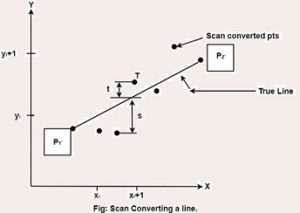Either the one to its right (lower-bound for the line). One top its right and up (upper-bound for the line) The line is best approximated by those pixels that fall the least distance from the path between P1′,P2′.

### Bresenham’s Line Algorithm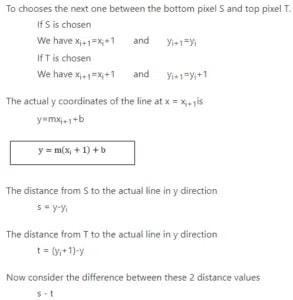When (s-t) <0 ⟹ s < t

The closest pixel is S

When (s-t) ≥0 ⟹ s < t

The closest pixel is T

This difference is
s-t = (y-yi)-[(yi+1)-y]
= 2y – 2yi -1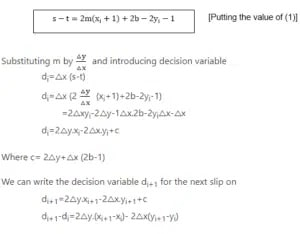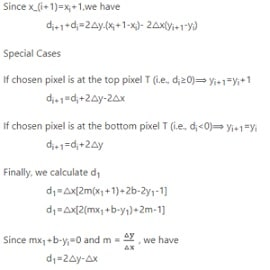1. It involves only integer arithmetic, so it is simple.

2. It avoids the generation of duplicate points.

3. It can be implemented using hardware because it does not use multiplication and division.

4. It is faster as compared to DDA (Digital Differential Analyzer) because it does not involve floating point calculations like DDA Algorithm.

1. This algorithm is meant for basic line drawing only Initializing is not a part of Bresenham’s line algorithm. So to draw smooth lines, you should want to look into a different algorithm.

### Bresenham’s Line Algorithm:

Step1: Start Algorithm

Step2: Declare variable x1,x2,y1,y2,d,i1,i2,dx,dy

Step3: Enter value of x1,y1,x2,y2
Where x1,y1are coordinates of starting point
And x2,y2 are coordinates of Ending point

Step4: Calculate dx = x2-x1
Calculate dy = y2-y1
Calculate i1=2*dy
Calculate i2=2*(dy-dx)
Calculate d=i1-dx

Step5: Consider (x, y) as starting point and xendas maximum possible value of x.
If dx < 0
Then x = x2
y = y2
xend=x1
If dx > 0
Then x = x1
y = y1
xend=x2

Step6: Generate point at (x,y)coordinates.

Step7: Check if whole line is generated.
If x > = xend
Stop.

Step8: Calculate co-ordinates of the next pixel
If d < 0
Then d = d + i1
If d ≥ 0
Then d = d + i2
Increment y = y + 1

Step9: Increment x = x + 1

Step10: Draw a point of latest (x, y) coordinates

Step11: Go to step 7

Step12: End of Algorithm

### Example: Starting and Ending position of the line are (1, 1) and (8, 5). Find intermediate points.

Solution: x1=1
y1=1
x2=8
y2=5
dx= x2-x1=8-1=7
dy=y2-y1=5-1=4
I1=2* ∆y=2*4=8
I2=2*(∆y-∆x)=2*(4-7)=-6
d = I1-∆x=8-7=1

 x y d=d+I1 or I2 1 1 d+I2=1+(-6)=-5 2 2 d+I1=-5+8=3 3 2 d+I2=3+(-6)=-3 4 3 d+I1=-3+8=5 5 3 d+I2=5+(-6)=-1 6 4 d+I1=-1+8=7 7 4 d+I2=7+(-6)=1 8 5

### Bresenham’s Line Algorithm

Program to implement Bresenham’s Line Drawing Algorithm:

#include<stdio.h>
#include<graphics.h>
void drawline(int x0, int y0, int x1, int y1)
{
int dx, dy, p, x, y;
dx=x1-x0;
dy=y1-y0;
x=x0;
y=y0;
p=2*dy-dx;
while(x<x1)
{
if(p>=0)
{
putpixel(x,y,7);
y=y+1;
p=p+2*dy-2*dx;
}
else
{
putpixel(x,y,7);
p=p+2*dy;}
x=x+1;
}
}
int main()
{
int gdriver=DETECT, gmode, error, x0, y0, x1, y1;
initgraph(&gdriver, &gmode, "c:\\turboc3\\bgi");
printf("Enter co-ordinates of first point: ");
scanf("%d%d", &x0, &y0);
printf("Enter co-ordinates of second point: ");
scanf("%d%d", &x1, &y1);
drawline(x0, y0, x1, y1);
return 0;
}

Output: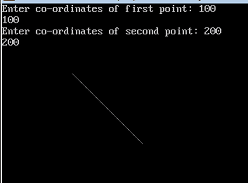Bresenham’s Line Algorithm

### Differentiate between DDA Algorithm and Bresenham’s Line Algorithm:

DDA Algorithm Bresenham’s Line Algorithm
1. DDA Algorithm use floating point, i.e., Real Arithmetic. 1. Bresenham’s Line Algorithm use fixed point, i.e., Integer Arithmetic
2. DDA Algorithms uses multiplication & division its operation 2.Bresenham’s Line Algorithm uses only subtraction and addition its operation
3. DDA Algorithm is slowly than Bresenham’s Line Algorithm in line drawing because it uses real arithmetic (Floating Point operation) 3. Bresenham’s Algorithm is faster than DDA Algorithm in line because it involves only addition & subtraction in its calculation and uses only integer arithmetic.
4. DDA Algorithm is not accurate and efficient as Bresenham’s Line Algorithm. 4. Bresenham’s Line Algorithm is more accurate and efficient at DDA Algorithm.
5.DDA Algorithm can draw circle and curves but are not accurate as Bresenham’s Line Algorithm, it can draw circle and curves with more accurate.

### Mid-Point Algorithm

Mid-point algorithm is due to Bresenham which was modified by Pitteway and Van Aken. Assume that you have already put the point P at x,yx,y coordinate and the slope of the line is 0 ≤ k ≤ 1 as shown in the following illustration. Now you need to decide whether to put the next point at E or N. This can be chosen by identifying the intersection point Q closest to the point N or E. If the intersection point Q is closest to the point N then N is considered as the next point; otherwise E.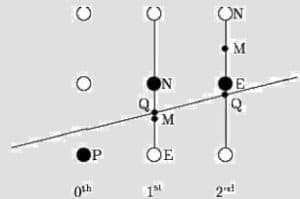To determine that, first calculate the mid-point Mx+1,y+½x+1,y+½. If the intersection point Q of the line with the vertical line connecting E and N is below M, then take E as the next point; otherwise take N as the next point. In order to check this, we need to consider the implicit equation:

Fx,yx,y = mx + b – y

For positive m at any given X,

• If y is on the line, then Fx,yx,y = 0
• If y is above the line, then Fx,yx,y < 0
• If y is below the line, then Fx,yx,y > 0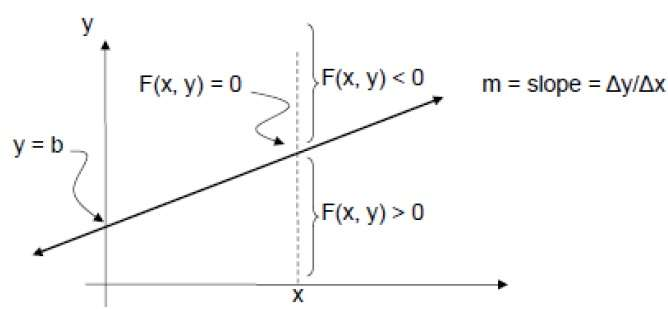## Laravel – Scopes (3 Easy Steps)

Scoping is one of the superpowers that eloquent grants to developers when querying a model. Scopes allow developers to add constraints to queries for a given model. In simple terms laravel scope is just a query, a query to make the code shorter and faster. We can...

## CAMBRIDGE IELTS 17 TEST 3

READING PASSAGE 1: The thylacine Q1. carnivorous keywords: Looked like a dog had series of stripes ate, diet ate an entirely 1 .......................................... diet (2nd paragraph 3rd and 4th line) 1st and 2nd paragraph, 1st  paragraph,resemblance to a...

## CAMBRIDGE IELTS 17 TEST 4

PASSAGE 1 Q1 (False) (Many Madagascan forests are being destroyed by attacks from insects.) Madagascar's forests are being converted to agricultural land at a rate of one percent every year. Much of this destruction is fuelled by the cultivation of the country's main...

## Cambridge IELTS 16 Test 4

Here we will discuss pros and cons of all the questions of the passage with step by step Solution included Tips and Strategies. Reading Passage 1 –Roman Tunnels IELTS Cambridge 16, Test 4, Academic Reading Module, Reading Passage 1 Questions 1-6. Label the diagrams...

## Cambridge IELTS 16 Test 2

Reading Passage 1: The White Horse of Uffington, Solution with Answer Key The White Horse of Uffington IELTS Cambridge 16, Test 2, Academic Reading Module, Reading Passage 1 Cambridge IELTS 16, Test 2: Reading Passage 1 – The White Horse of Uffington...

# You may find interest following article

## Laravel – Scopes (3 Easy Steps)

Scoping is one of the superpowers that eloquent grants to developers when querying a model. Scopes allow developers to add constraints to queries for a given model. In simple terms laravel scope is just a query, a query to make the code shorter and faster. We can create custom query with relation or anything with scopes. In any admin project we need to get data...

## CAMBRIDGE IELTS 17 TEST 3

READING PASSAGE 1: The thylacine Q1. carnivorous keywords: Looked like a dog had series of stripes ate, diet ate an entirely 1 .......................................... diet (2nd paragraph 3rd and 4th line) 1st and 2nd paragraph, 1st  paragraph,resemblance to a dog. … dark brown stripes over its back, beginning at the rear of the body and extending onto the...

## CAMBRIDGE IELTS 17 TEST 4

PASSAGE 1 Q1 (False) (Many Madagascan forests are being destroyed by attacks from insects.) Madagascar's forests are being converted to agricultural land at a rate of one percent every year. Much of this destruction is fuelled by the cultivation of the country's main staple crop: rice. And a key reason for this destruction is that insect pests are destroying vast...

## Cambridge IELTS 16 Test 4

Here we will discuss pros and cons of all the questions of the passage with step by step Solution included Tips and Strategies. Reading Passage 1 –Roman Tunnels IELTS Cambridge 16, Test 4, Academic Reading Module, Reading Passage 1 Questions 1-6. Label the diagrams below. The Persian Qanat Method 1. ………………………. to direct the tunnelingAnswer: posts – First...

## Cambridge IELTS 16 Test 3

Reading Passage 1: Roman Shipbuilding and Navigation, Solution with Answer Key , Reading Passage 1: Roman Shipbuilding and Navigation IELTS Cambridge 16, Test 3, Academic Reading Module Cambridge IELTS 16, Test 3: Reading Passage 1 – Roman Shipbuilding and Navigation with Answer Key. Here we will discuss pros and cons of all the questions of the...

## Cambridge IELTS 16 Test 2

Reading Passage 1: The White Horse of Uffington, Solution with Answer Key The White Horse of Uffington IELTS Cambridge 16, Test 2, Academic Reading Module, Reading Passage 1 Cambridge IELTS 16, Test 2: Reading Passage 1 – The White Horse of Uffington  with Answer Key. Here we will discuss pros and cons of all the questions of the passage with...

## Cambridge IELTS 16 Test 1

Cambridge IELTS 16, Test 1, Reading Passage 1: Why We Need to Protect Bolar Bears, Solution with Answer Key Cambridge IELTS 16, Test 1: Reading Passage 1 – Why We Need to Protect Bolar Bears with Answer Key. Here we will discuss pros and cons of all the questions of the passage with step by step...

PASSAGE 1: THE RETURN OF THE HUARANGO QUESTIONS 1-5: COMPLETE THE NOTES BELOW. 1. Answer: water Key words:  access, deep, surface Paragraph 2 provides information on the role of the huarango tree: “it could reach deep water sources”. So the answer is ‘water’. access = reach Answer: water. 2. Answer: diet Key words: crucial,...

PASSAGE 1: HENRY MOORE (1898 – 1986 ) QUESTIONS 1-7: DO THE FOLLOWING STATEMENTS AGREE WITH THE INFORMATION GIVEN IN READING PASSAGE 1? 1. Answer: TRUE Key words: leaving school, Moore, did, father, wanted It is mentioned in the first paragraph that “After leaving school, Moore hoped to become a sculptor, but instead he complied with his father’s...

PASSAGE 1: COULD URBAN ENGINEERS LEARN FROM DANCE ?  QUESTIONS 1- 6: READING PASSAGE 1 HAS SEVEN PARAGRAPHS, A-G. 1. Answer: B Key words: way of using dance, not proposing By using the skimming and scanning technique, we would find that before going into details about how engineers can learn from dance, the author first briefly mentions ways of...

PASSAGE 1: NUTMEG – A VALUABLE SPICE QUESTIONS 1- 4: COMPLETE THE NOTES BELOW.CHOOSE ONE WORD ONLY FROM THE PASSAGE FOR EACH ANSWER.WRITE YOUR ANSWER IN BOXES 1-8 ON YOUR ANSWER SHEET. 1. Answer: oval Key words: leaves, shape Using the scanning skill, we can see that the first paragraph describes the characteristics of the tree in detail, including...

PASSAGE 1: THE SECRET OF STAYING YOUNG QUESTIONS 1-8: COMPLETE THE NOTES BELOW. 1. ANSWER: FOUR / 4 Explain– Key words: focused age groups, ants– In paragraph 3, it is stated that “Giraldo focused on ants at four age ranges”,so the answer must be “four/4”. 2. ANSWER: YOUNG Explain– Key words: how well, ants, looked after– The first sentence of...

PASSAGE 1: THE CONCEPT OF INTELLIGENCE QUESTIONS 1-3: READING PASSAGE 1 HAS SIX PARAGRAPHS, A-F. 1. ANSWER: B Explain ·     Key words: non-scientists, assumptions, intelligence, influence, behavior ·    People‟s behavior towards others‟ intelligence is mentioned in the first sentence of paragraph B: “implicit theories of...

Cambridge IELTS 14 is the latest IELTS exam preparation.https://draftsbook.com/ will help you to answer all questions in cambridge ielts 14 reading test 2 with detail explanations. PASSAGE 1: ALEXANDER HENDERSON (1831-1913) QUESTIONS 1-8: DO THE FOLLOWING STATEMENTS AGREE WITH THE INFORMATION GIVEN IN READING PASSAGE 1? 1. ANSWER: FALSE Explain Henderson rarely...

Cambridge IELTS 14 is the latest IELTS exam preparation.https://draftsbook.com/ will help you to answer all questions in cambridge ielts 14 reading test 1 with detail explanations. PASSAGE 1: THE IMPORTANCE OF CHILDREN’S PLAY QUESTIONS 1-8: COMPLETE THE NOTES BELOW. 1. ANSWER: CREATIVITY Explain building a “magical kingdom” may help develop … – Key words: magical...

PASSAGE 1: CUTTY SARK: THE FASTEST SAILING SHIP OF ALL TIME QUESTIONS 1-8: DO THE FOLLOWING STATEMENTS AGREE WITH THE INFORMATION GIVEN IN READING PASSAGE 1? 1. CLIPPERS WERE ORIGINALLY INTENDED TO BE USED AS PASSENGER SHIPS Key words: clippers, originally, passengerAt the beginning of paragraph 2, we find the statement: “The fastest commercial sailing...

Cambridge IELTS 13 is the latest IELTS exam preparation.https://draftsbook.com/ will help you to answer all questions in cambridge ielts 13 reading test 3 with detail explanations. PASSAGE 1: THE COCONUT PALM QUESTIONS 1-8: COMPLETE THE TABLE BELOW. 1.TRUNK – TIMBER FOR HOUSES AND THE MAKING OF………. Key words: trunk, timber, houses, makingLooking for...